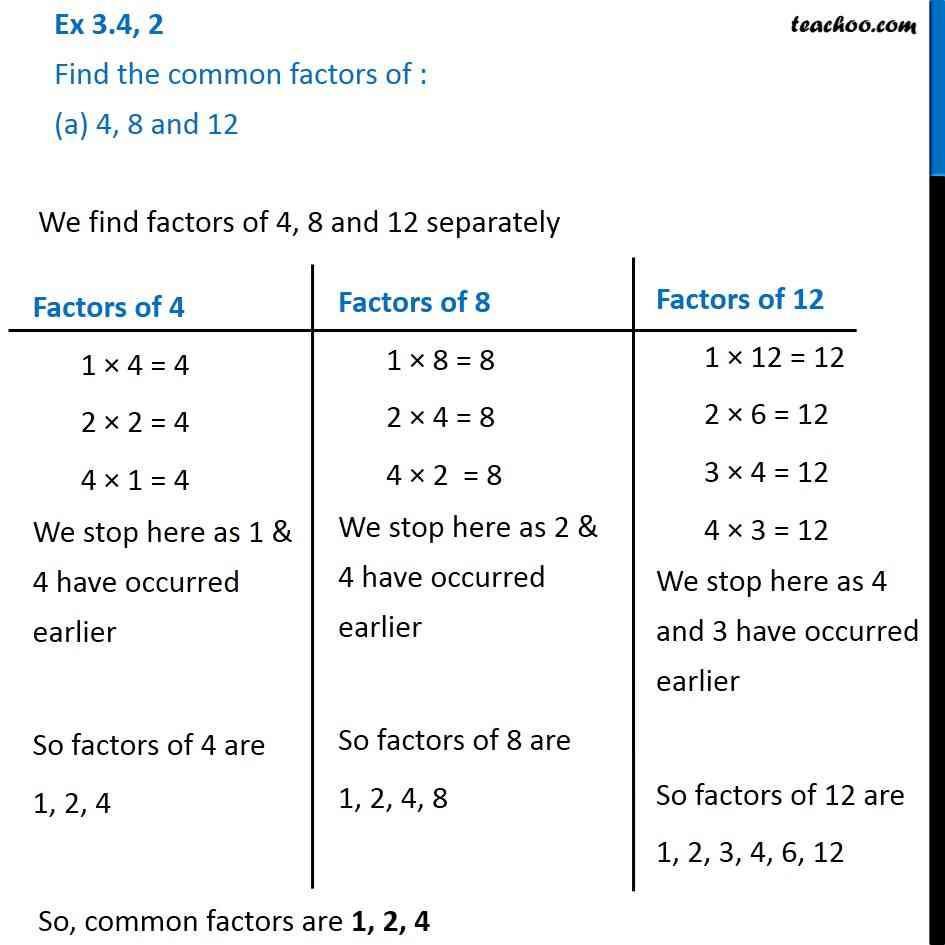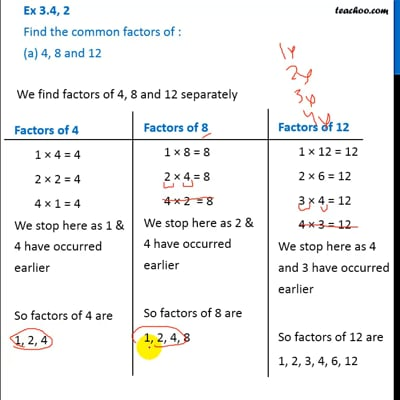Ex 3.4

Chapter 3 Class 6 Playing with Numbers
Serial order wiseThis video is only available for Teachoo black users

### Transcript

Ex 3.4, 2 Find the common factors of : (a) 4, 8 and 12 We find factors of 4, 8 and 12 separately So, common factors are 1, 2, 4 Factors of 4 1 × 4 = 4 2 × 2 = 4 4 × 1 = 4 We stop here as 1 & 4 have occurred earlier So factors of 4 are 1, 2, 4 Factors of 8 1 × 8 = 8 2 × 4 = 8 4 × 2 = 8 We stop here as 2 & 4 have occurred earlier So factors of 8 are 1, 2, 4, 8 Factors of 12 1 × 12 = 12 2 × 6 = 12 3 × 4 = 12 4 × 3 = 12 We stop here as 4 and 3 have occurred earlier So factors of 12 are 1, 2, 3, 4, 6, 12